#Interactive Real Analysis

Next | Previous | Glossary | Map

## 6.6. A Function Primer

### Cantor Function

The Cantor function is a function that is continuous, differentiable, increasing, non-constant, and the derivative is zero everywhere except at a set with length zero.
This is the most difficult function in our repertoire and can be found, for example, in Kolmogorov and Fomin.

Recall the definition of the Cantor set: Let= [ 1/3, 2/3]
be the middle third of the interval [0, 1]. Let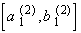= [ 1/9, 2/9],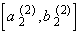= [ 7/9, 8/9 ]
be the middle thirds of the intervals remaining after deletingfrom [0, 1]. Let= [2/27, 3/27],= [7/27, 8/27],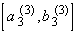= [19/27, 20/27],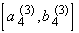= [ 25/27, 26,27]
be the middle thirds of the intervals remaining after deleting,, andfrom [0, 1]. Continue in this fashion so that at the n-th stage we have the intervals, ...,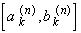, ...,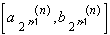Then the complement of the union of all these intervalsis the Cantor set without the endpoints. Now define the following functionif t is in the intervalThen, for example, we have that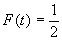if 1/3t2/3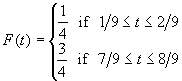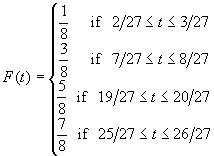Then F(t) is defined everywhere in [0, 1] except at the Cantor set minus the end points 0, 1, 1/3, 2/3, 1/9, 2/9, 7/9, 8/9, ... If t is a number where F is not defined, then there exists an increasing sequence { xn } of these endpoints converging to t, and a decreasing sequence { xn' } of these endpoints converging to t. Since F is defined at those endpoints xn and xn', we define

F(t) =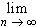F(xn) =F(xn')

Now we have defined completely the Cantor function. It has the following properties:

• F is defined everywhere in the interval [0, 1]
• F is not constant
• F is increasing
• F is continuous in the interval [0, 1]
• F is differentiable in the interval [0, 1]
• F' is zero at every interior point of the intervalsIn particular, F' is zero at points of total length 1 in the interval [0, 1], yet F it is not constant.

### Proof

Some of these properties are obvious, and some require more thought. In particular, why does the above limit of endpoints exist ? That is a crucial point, because we used this limit to extend the function to the whole interval [0, 1]. For details and hints about the Cantor Function, please consult Kolmogorov and Fomin, p 334 ff.

Next | Previous | Glossary | Map